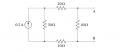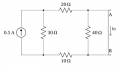# Finding Thevenin Equivalent - single current source

#### Andy1987

Joined Apr 26, 2015
2
Hi, I am revising for an exam and I am having problems finding thevenin equivalent circuits when I am given a circuit with a single current source. I am usually fine at solving these type of questions when I'm given a voltage source. My lecturer did not go through any examples of how to solve these circuits, So i would greatly appreciate some advice on this.My attempt at the problem so far:

I am able to find the Thevenin / Norton resistance by:

Open the current source, then Rth is given by (30+20+10)// 40 = 24 ohm

Now I am just stuck as to how I should find the Vth. I know the answer is 6v. Really appreciate some help with these type of problems . Thanks!

#### MrAl

Joined Jun 17, 2014
10,925
Hi,

There are other ways to do this too, working left to right, right to left, right to left then left to right, or as you did with the resistance first.
In that case you should next short the output to obtain the short circuit current, which then you can apply to your resistance.

You could check your result by using a Thev/Norton collapse of the network from left to right and see if you get the same result. To collapse from left to right, use Thev then Norton, then Thev then Norton, then Thev then Norton, etc., alternating until you get to the right side of the network.

#### Andy1987

Joined Apr 26, 2015
2
After looking at it some more, I think If i Look at the current source, some current will flow through the 30ohm, and the rest through a series of 20+40+10 = 70ohm, so I can use the current divider to find the current in the 70ohm series is 0.15A, Vth = 0.15*40 = 6V.

In terms of shorting the circuit - i looked at this, but I can't figure out what happens when I do that. Would you mind explaining what happens when we short it across AB? Cheers for your help

#### WBahn

Joined Mar 31, 2012
29,521
If you were just given this circuit and asked to find the voltage between A and B, could you? Forget about Thevenin equivalents, it's just a circuit they type of which you have been analyzing since shortly after the beginning of the course. Don't make it harder than it is.

As for finding the short circuit current, consider the following circuit:If asked to find Io in this circuit, could you do it?

#### MrAl

Joined Jun 17, 2014
10,925
After looking at it some more, I think If i Look at the current source, some current will flow through the 30ohm, and the rest through a series of 20+40+10 = 70ohm, so I can use the current divider to find the current in the 70ohm series is 0.15A, Vth = 0.15*40 = 6V.

In terms of shorting the circuit - i looked at this, but I can't figure out what happens when I do that. Would you mind explaining what happens when we short it across AB? Cheers for your help
Hello again,

Well yes, but now you are doing it a different way. As i mentioned, there are several ways to do this and your new method is another way also, but because you first found the resistance i assumed you had to do it that way.

The whole idea with a linear network is to find the equivalent voltage source and series resistance (see picture 1) and you already found the resistance. The more general way to find the resistance is to first find the short circuit current and then the open circuit voltage. then divide that voltage by that current. So say you got 2 amps and 5 volts, then the resistance Rs would be 5/2=2.5 ohms. The open circuit voltage is easy because you just analyze the network, and that would be 5 volts, so Vs=5 and Rs=2.5 for that network.

Your network as a few more resistors so you have to do a little more work, but if you short out the network (see WBahn's drawing in the previous post) you'll get the short circuit current, and if you just use a little analysis you'll get the open circuit voltage, and then divide for the resistance Rs and you have it.

The main theories of Thevenin and Norton are perhaps better stated like this:
1. A current source in parallel with a resistance is equivalent to a voltage source in series with a resistance.
2. A voltage source in series with a resistance is equivalent to a current source in parallel with a resistance.

If we apply the short circuit current and open circuit voltage idea to these two statements for the first picture below, to get the bottom circuit from the top circuit we just have to short the network and calculate the current through the short, then open circuit the network and calculate the voltage.

For the top network shown and Is=1 and Rp=2, the open circuit voltage is 2*1=2 volts, and the short circuit current is 1, so the resistance Rs=2/1=2 ohms. The open circuit voltage is 2 volts so the Thevenin voltage is 2 volts.
For the bottom network shown and Vs=2 and Rs=2, the open circuit voltage is 2 volts, and the short circuit current is 2/2=1 amp, so the resistance is 2/1=2 ohms so Rp=2 ohms. The short circuit current is 1 amp so the Norton current is 1 amp.
Note the similarities between these two networks and that's what the two theorems are really telling us.

In more complex networks we can use these principles to simplify the network by using them one or more times as needed. Your network and the equivalent we need are shown in picture 2. The most notable thing here is that when we short either network we get the same short circuit current (through the short itself placed across AB), and when we open circuit both networks we get the same open circuit voltage (across AB).

#### Dodgydave

Joined Jun 22, 2012
11,151
whats the 0.5Amp source doing,is it flowing through the 30 ohms or is it just for decoration!

#### WBahn

Joined Mar 31, 2012
29,521
whats the 0.5Amp source doing,is it flowing through the 30 ohms or is it just for decoration!
Neither.

#### MrAl

Joined Jun 17, 2014
10,925
Hi,

WBahn:
That might be the most accurate and correct single word answer i've ever readDodgydave:
Current tends to flow in any path it can possibly get to, with the exception of a perfectly zero ohm short circuit where all the current flows through the short. In his circuit there are two paths, so part will flow in one branch and part in the other and if the resistances in those branches are different then the current sharing will mean a different current in each branch. If the resistances are equal then half the current will flow in each branch if there are two branches.